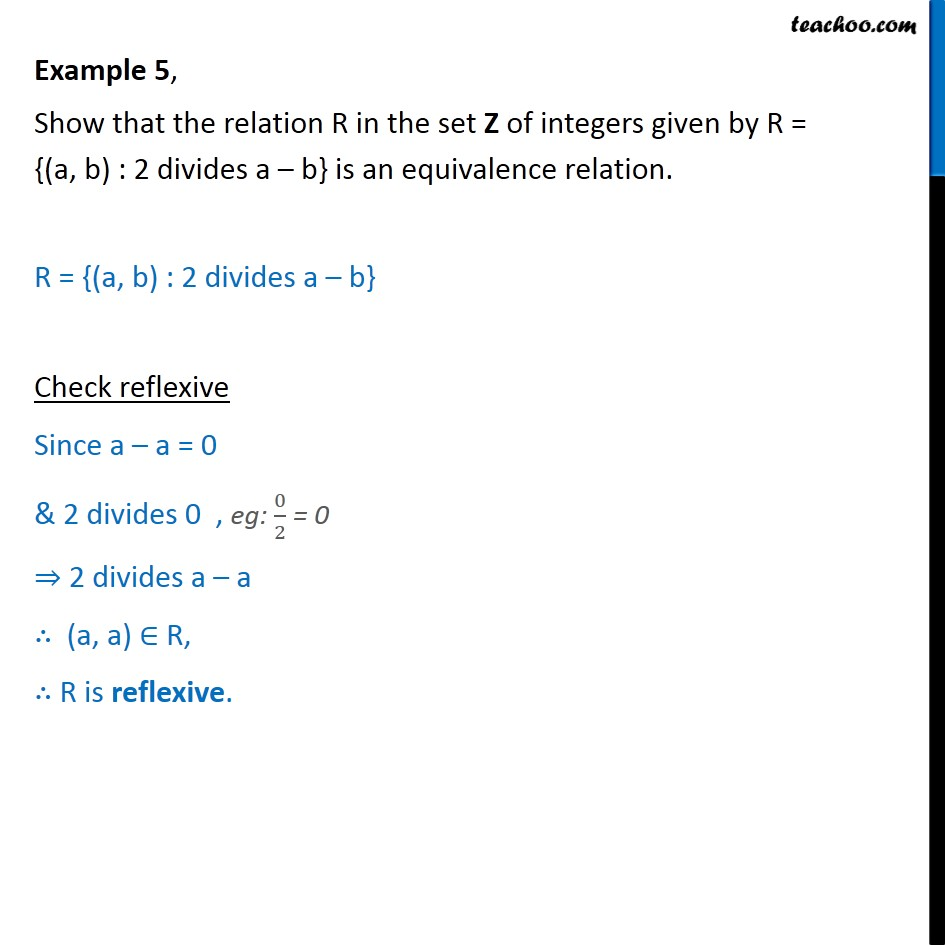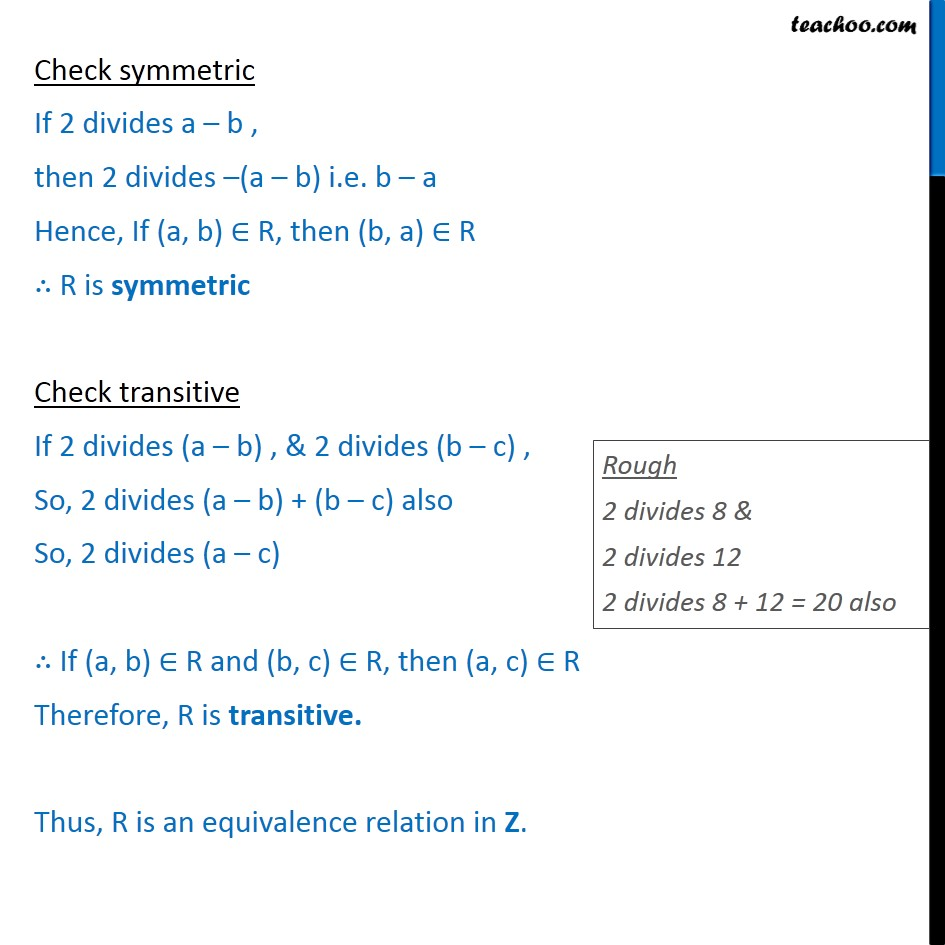To prove relation reflexive, transitive, symmetric and equivalent

Chapter 1 Class 12 Relation and Functions
Concept wiseIntroducing your new favourite teacher - Teachoo Black, at only ₹83 per month

### Transcript

Example 5, Show that the relation R in the set Z of integers given by R = {(a, b) : 2 divides a b} is an equivalence relation. R = {(a, b) : 2 divides a b} Check reflexive Since a a = 0 & 2 divides 0 , eg: 0 2 = 0 2 divides a a (a, a) R, R is reflexive. Check symmetric If 2 divides a b , then 2 divides (a b) i.e. b a Hence, If (a, b) R, then (b, a) R R is symmetric Check transitive If 2 divides (a b) , & 2 divides (b c) , So, 2 divides (a b) + (b c) also So, 2 divides (a c) If (a, b) R and (b, c) R, then (a, c) R Therefore, R is transitive. Thus, R is an equivalence relation in Z.